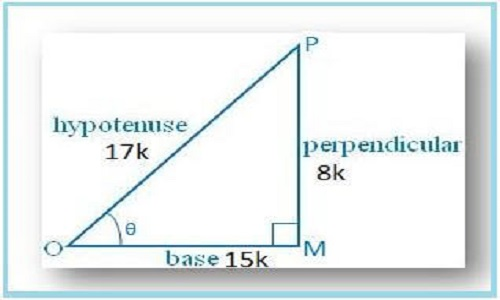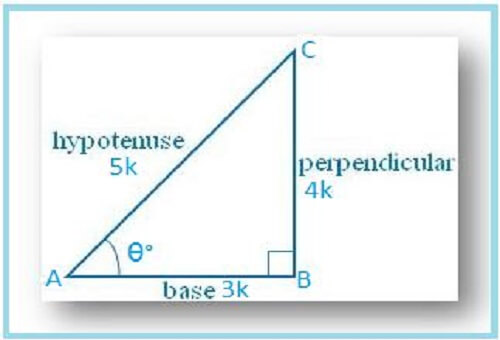## Mathematics tutors

Our IB Maths Tutors say that Trigonometry is a very important and interesting branch of Maths

Trigonometry is one of the branches of Mathematics that fascinates Mathematicians. It tells about the relationships between the angles and sides of a triangle. Word trigonometry was started from the Greek word, where,

TRI‘ = Three

‘GON‘= sides and the ‘METRON’ = to measure. It’s an ancient and probably most widely applicable branch in Mathematics.

### Parts of Trigonometry-

For basic learning, I am dividing trigonometry in two-part:-

1 Trigonometry based on right triangles

1 Trigonometry based on non-right triangles.

In this post, I will only discuss Trigonometry based on right triangles.

In a right triangle, there are three sides hypotenuse (the longest side), adjacent side(base) and the opposite side(perpendicular).

### Pythagoras theorem

If we know any two sides of the right triangle, we can find the third side by using Pythagoras theorem:-

Also READ this-  Pi day-14th March
##### P²+B²=H²

In a right triangle, the ratios of sides can be written in many ways like P/H, B/H, P/B, H/P, H/B, B/P. each ratio is called a trigonometric ratio and these are represented by Latin words Sin, Cos, Tan, Cosec, Sec and Cot.
In a right triangle

Sin =P/H, Cos =B/H,  Tan= P/B, Cosec= H/P, Sec =H/B, Cot= B/P,

Mathematics tutors use these three concepts to solve trigonometric problems

Concept-1

In a trigonometric problem, If we are given a trigonometric ratio or asked about it, we will first find all three sides of the triangle

#### Sample Problems

Example-1  If sin θ = 8/17, find other trigonometric ratios of θ.

Ans-

Let us draw a ∆ OMP in which ∠M = 90°Also READ this-  How To Solve Limit Problems

Then sin θ = MP/OP = 8/17.

Let MP = 8k and OP = 17k, where k is positive.

By Pythagoras’ theorem, we get

OP2 = OM2 + MP2

⇒ OM2 = OP2 – MP2

⇒ OM2 = [(17k)2 – (8k)2]

⇒ OM2 = [289k2 – 64k2]

⇒ OM2 = 225k2

⇒ OM = √(225k2)

⇒ OM = 15k

Therefore, sin θ = MP/OP = 8k/17k = 8/17

cos θ = OM/OP = 15k/17k = 15/17

tan θ = Sin θ/Cos θ = (8/17 × 17/15) = 8/15

csc θ = 1/sin θ = 17/8

sec θ = 1/cos θ = 17/15 and

cot θ = 1/tan θ = 15/8.

Example-2 If cos θ = 3/5, find the value of (5cosec θ – 4 tan θ)/(sec θ + cot θ)

Let us draw an ∆ ABC in which ∠B = 90°.Let ∠A = θ°

Then cos θ = AB/AC = 3/5.

Let AB = 3k and AC = 5k, where k is positive.

By Pythagoras’ theorem, we get

AC2 = AB2 + BC2

⇒ BC2 = AC2 – AB2

⇒ BC2 = [(5k)2 – (3k)2]

Also READ this-  Continuity of functions | Learn Maths Online

⇒ BC2 = [25k2 – 9k2]

⇒ BC2 = 16k2

⇒ BC = √(16k2)

⇒ BC = 4k

Therefore, sec θ = 1/cos θ = 5/3

tan θ = BC/AB =4k/3k = 4/3

cot θ = 1/tan θ = 3/4 and

csc θ = AC/BC = 5k/4k = 5/4

Now (5cosec θ -4 tan θ)/(sec θ + cot θ)

= (5 × 5/4 – 4 × 4/3)/(5/3 + 3/4)

= (25/4 -16/3)/(5/3 +3/4)

= 11/12 × 12/29

= 11/29

In next post of Mathematics tutors series, we will discuss the remaining two concepts of non-right triangle trigonometry

To get a better understanding of the concept you should check all my posts of Mathematics tutors series. you can check them by clicking on the links given below

#### Fourth post -Concept four